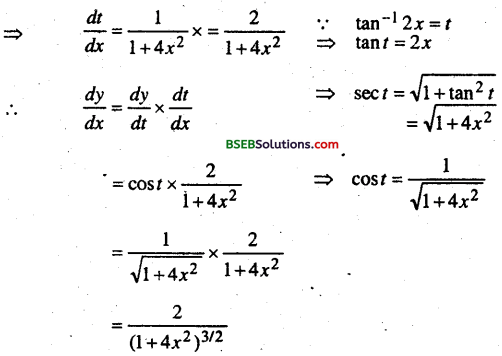# Bihar Board 12th Maths Important Questions Short Answer Type Part 1

BSEB Bihar Board 12th Maths Important Questions Short Answer Type Part 1 are the best resource for students which helps in revision.

## Bihar Board 12th Maths Important Questions Short Answer Type Part 1

Relation & Functions

Question 1.
Prove that the function f :R→R given by f(x) = 2x is one-one & on to.
Solution:
For one-one – Take f(x1) = f(x2) .
⇒ 2x1 = 2x2
⇒ x1 = x2
∴ f is one-one.
For on to co-domain = R
Rage = y = 2x = 2 x R = R
∴ Co-domain = Rage
∴ f is on to
Hence f is one-one onto.Question 2.
Show that the function f: N → N given by f(1) = f(2) = 1 & f(x) = x-1 for every x > 2 is on to but not one-one,
Solution:
∴ f(1) = f(2) = 1
∴ f is not one-one
∴ f(x) = x – 1
∵ Co -domain = N
∀ x > 2, Rage = N
∴ Co-domain = Rage
∴ f(x) is on to.Question 3.
Let L be the set of all lines in a plane and# he the relation inL defined as R = { (L1 L2) : L1 is perpendicular to L2). Show that R is symmetric but neither reflexive nor transitive.
a line L1 can not be ⊥r to itself.
R is not reflexive.
For symmetric — If L1 RL2
⇒ L2RL1⇒ L1 is ⊥r to L2
⇒ L2 is ⊥r to L1
⇒ (L2,L1) ∈ R.
∴ R is symmetric
for transitive — If L1 RL2, L2RL3 = L1RL3
Given is ⇒ L1 is ⊥r L2
& . L2 is lr L3
⇒ L1||L3
⇒ L1 is ⊥r L2
⇒ L2 is ⊥r L3
⇒ L1||L3
∴ R is not transitive.Question 4.
Show that the function f:R→R: f(x) =x3 is one-one and onto.
Solution:
Given, f(x1) = f(x2) ⇒ x13 = x23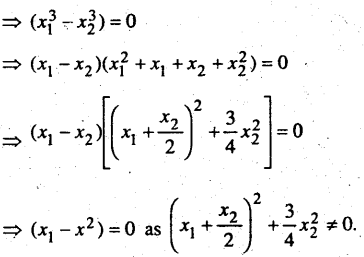⇒ x1 = x2
⇒ f is one-one.
Let y ∈ R and 3; = x3; Then x = y1/3 e R.
Thus, for each y in the codonain R there exists y1/3 in R such that
f(y1/3) = (y1/3)3 = y
⇒ f is onto.
Hence, f is one-one to.

Matrix

Question 1.
If A = $$\left[\begin{array}{cc} 2 & 4 \\ 3 & 2 \end{array}\right]$$, B = $$\left[\begin{array}{ll} 1 & 3 \\ -2 & 5 \end{array}\right]$$ , C = $$\left[\begin{array}{rr} -2 & 5 \\ 3 & 4 \end{array}\right]$$ then find the value of (i) A- B (ii) 3A – C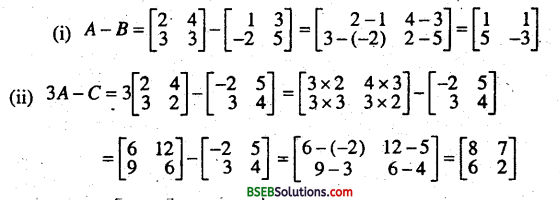Question 2.
If A = $$\left[\begin{array}{rr} 3 & 1 \\ -1 & 2 \end{array}\right]$$ Prove that A2 – 5. A + 7I= 0.
Solution: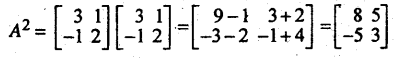Question 3.
If Aα = $$\left[\begin{array}{rr} \cos \alpha & \sin \alpha \\ -\sin \alpha & \cos \alpha \end{array}\right]$$, Prove that AαAβ = AβAα = Aα+β
Solution: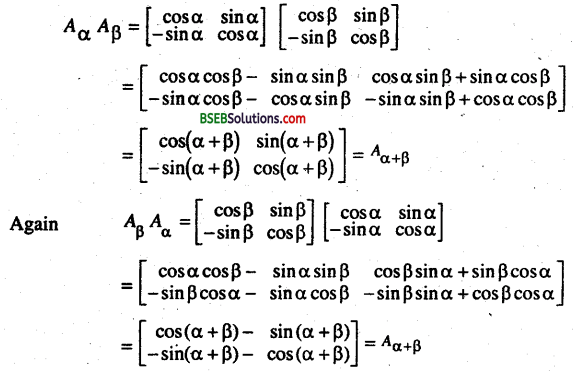AαAβ = AβAα = Aα+βQuestion 4.
If A = $$\left[\begin{array}{ll} 3 & 2 \\ 1 & 1 \end{array}\right]$$
Find the number a and b such that A2 + aA + bI = 0 where I is an identity matrix of order 2×2 and 0 is a 2 x 2 Zero matrix.
Solution:Equation the respective elements of two matrices we get.
8 + 2a = 0 ⇒ a =—4
and 11 + 3a + b = 0 ⇒ 11 – 12 + b = 0 ⇒ b= 1
Hence .
a = -4, b = 1.

Determinants

Question 1.
Prove that $$\left|\begin{array}{ccc} a+b+2 c & a & b \\ c & b+c+2 a & b \\ c & a & c+a+2 b \end{array}\right|$$ = 2(a + b + c)3
Solution:
Operating C1 + C2 + C3 is the determinant of L.H.S.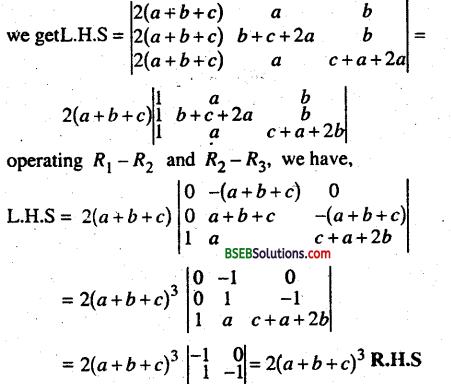Question 2.
Find the area of the triangle with vertices (-2,-3), (3,2) and (-1,-8).
Area of the triangle = $$\frac{1}{2}\left|\begin{array}{lll} x, & y, & 1 \\ x_{2} & y_{2} & 1 \\ x_{3} & y_{3} & 1 \end{array}\right|$$
= $$\frac{1}{2}\left|\begin{array}{rrr} -2 & -3 & 1 \\ 3 & 2 & 1 \\ -1 & -8 & 1 \end{array}\right|=\frac{1}{2}\left|\begin{array}{rrr} -5 & -5 & 0 \\ 4 & 10 & 0 \\ -1 & -8 & 1 \end{array}\right|$$ (R1 – R2, R2 – R3
= – 15 = 15 5q.units.Question 3.
Find Values of k if area of triangle is 4 Sq. units and vertices are : (k, 0), (4,0)’, (0,2).
Solution:
Δ = $$\left|\begin{array}{lll} 1 & 1 & 1 \\ k & 4 & 0 \\ 0 & 0 & 2 \end{array}\right|$$ or, 4 = $$\left|\begin{array}{lll} 1 & 1 & 1 \\ k & 4 & 0 \\ 0 & 0 & 2 \end{array}\right|$$
or, 4 = 2$$\left|\begin{array}{ll} 1 & 1 \\ k & 4 \end{array}\right|$$ or, 4 = 2(4 – k)
or, 4 – k = 2 or, 4 = 2(4 – k)
or, 4 – k = 2 ∴ k = 2
4 – 2 = k

Inverse circular Function

Question 1.
Find the principal value of following :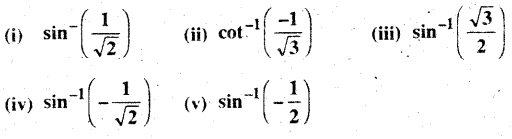Solution:
(i) Let sin-1([latex-\frac{1}{\sqrt{2}}][/latex]) = y
Then sin y = $$\frac{1}{\sqrt{2}}$$ ≠ +ve (1st or 2nd quadram)
Last value of Angle $$\frac{\pi}{4}$$
∴ P. V of $$\sin ^{-1}\left(\frac{1}{\sqrt{2}}\right)=\frac{\pi}{4}$$

(ii) Let y = cot-1([latex-\frac{1}{\sqrt{3}}][/latex]) or cot y = [latex-\frac{1}{\sqrt{3}}][/latex]
We know that the range of principal value of Cot-1 is (0, π)Hence P.V $$\frac{2 \pi}{3}$$

(iii) Ley y = sin-1 $$\frac{\sqrt{3}}{2}$$
then sin y = $$\frac{\sqrt{3}}{2}$$
∴ Range of Principal value of sin-1 is $$\left[\frac{-\pi}{2}, \frac{\pi}{2}\right]$$
∴ sin y = $$\frac{\sqrt{3}}{2} \sin \frac{\pi}{3}$$
∴ P.V $$\frac{2 \pi}{3}$$

(iv) Let y = sin-1 ( $$-\frac{1}{\sqrt{2}}$$ )
or sin y = $$-\frac{1}{\sqrt{2}}$$
∵ Range of P.V of sin-1 is $$\left[\frac{-\pi}{2}, \frac{\pi}{2}\right]$$
∴ sin y = sin $$-\frac{\pi}{4}$$
∴ P.V = $$-\frac{\pi}{4}$$

(v) sin y = sin-1 ($$-\frac{1}{2}$$)
∵ Range of P. V. of sin -1 is $$\left[\frac{-\pi}{2}, \frac{\pi}{2}\right]$$
∴ sin y = sin (-π/4)
∴ P.V = $$-\frac{\pi}{6}$$

Question 2.
Find the value of sin-1(sin $$\frac{3 \pi}{5}$$
Solution:
we knoe that,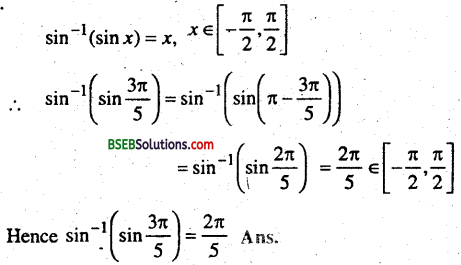Question 3.
Prove that $$2 \tan ^{-1} \frac{1}{3}+\tan ^{-1} \frac{1}{7}=\frac{\pi}{4}$$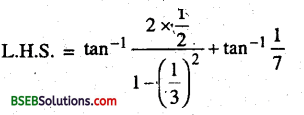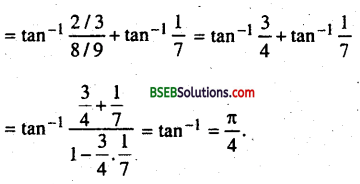Question 4.
Prove that $$\tan ^{-1} \frac{\alpha-\beta}{1+\alpha \beta}+\tan ^{-1} \frac{\beta-\gamma}{1+\beta \gamma}+\tan ^{-1} \frac{\gamma-\alpha}{1+\gamma \alpha}$$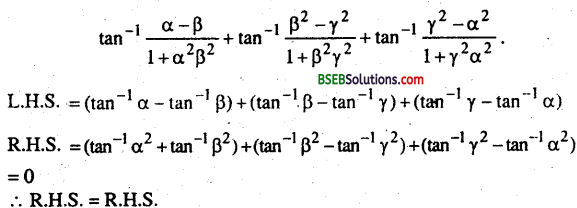Question 5.
Prove that $$\sin ^{-1} \frac{4}{5}+\sin ^{-1} \frac{5}{13}+\sin ^{-1} \frac{16}{25}=\frac{\pi}{2}$$Question 6.
Solve 2tan-1(cosx) = tan-1(2 cosec x)
2tan-1(cosx)
= $$\tan ^{-1}\left(\frac{2 \cos x}{1-\cos ^{2} x}\right)=\tan ^{-1}\left(\frac{2 \cos x}{\sin ^{2} x}\right)$$
Putting this valu in (1)
∴ $$\tan ^{-1}\left(\frac{2 \cos x}{\sin ^{2} x}\right)$$ = s cosec x = $$\frac{2}{\sin x}$$
cos x = sin x or tan x = 1
⇒ x = $$\frac{\pi}{4}$$

Question 7.
$$\tan ^{-1} \frac{1-x}{1+x}=\frac{1}{2} \tan ^{-1} x$$, x > 0
$$\frac{1}{2} \tan ^{-1} x=\tan ^{-1} \frac{1-x}{1+x}$$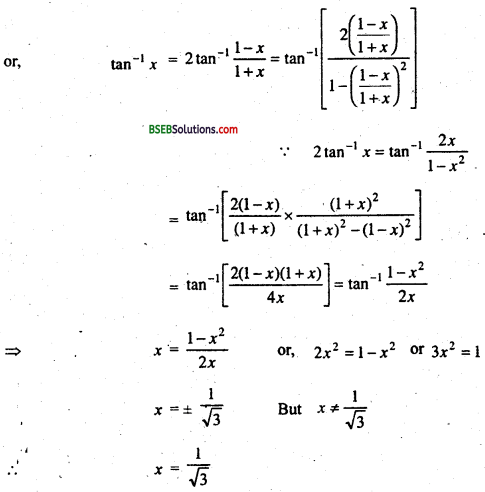Question 8.
sin-1(1 – x) – 2sin-1 x – $$\frac{\pi}{2}$$ then x is equal to
(A) 0, $$\frac{1}{2}$$
(B) 1, $$\frac{1}{2}$$
(C) 0
(D) $$\frac{1}{2}$$
Solution:
sin-1(1 – x) – 2sin-1 x – $$\frac{\pi}{2}$$
Putting $$\frac{\pi}{2}$$ = sin-1(1 – x) + cos-1 (1-x)
-2sin-1 = cos-1(x-1)
Let sin-1 = α
-2sin-1 = -2α = cos-1(x-1)
or cos 2α = 1 – x
1 – 2cos2α = (1 – x)
Putting sin α = x
1 – 2x2 = 1 – x
or 2x2-x = 0
x(2x – 1) = 0 x = 0, $$\frac { 1 }{ 2 }$$
But x = $$\frac { 1 }{ 2 }$$ does not satisfy the equation ∴ x = 0
∴ Part (C) is the correct answer.

Continuity and Differentiabiilty

Question 1.
f(x) = x sin $$\frac { 1 }{ x }$$ , x ≠ 0 and f(0) = 0.
Solution: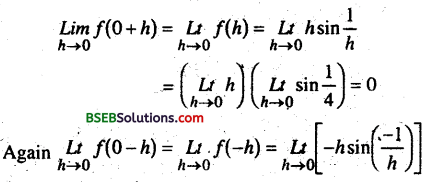= $$L_{h \rightarrow 0}\left(h \sin \frac{1}{4}\right)$$ = 0
Also given that f(0) = OSo, function is continous at x = 0

Question 2.
Prove that every differentiable function is continuous also.
Solution:
Let the function f(x) is differentiable at x = a.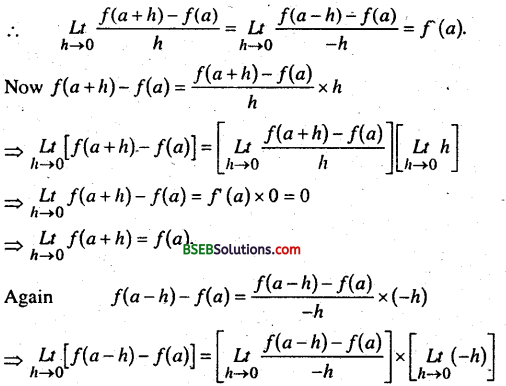$$\underset{h \rightarrow 0}{L t}$$ f(a -h) – f(a) = f1(a) x 0 = 0
$$\underset{h \rightarrow 0}{L t}$$ f(a – h) = f(a)
$$\underset{h \rightarrow 0}{L t}$$f(a + h) $$\underset{h \rightarrow 0}{L t}$$f(a
So, $$\underset{h \rightarrow 0}{L t}$$ f(a+h) = $$\underset{h \rightarrow 0}{L t}$$ f(a-h) = f(a)
∴ f(x) is continuous at x = a.Differentiation

Question 1.
Differentiate the following w.r.t to x.
(i) Cos(sin(log a))
(ii) log (x + $$\sqrt{a^{2}+x^{2}}$$
(iii) log $$\sqrt{\frac{1-\cos x}{1+\cos x}}$$
(iv) loge (logen
(v) ta-1$$\left(\frac{1-x}{1+x}\right)$$
(vi) sin(x2 + 1)
(vii) tan-1$$\frac{\sqrt{1+x^{2}}-1}{x}$$
(viii) (logx)logx
(ix) (x)x
(x) (logx)x + xlogx
(xi) cos (a cos x + b sin x)
(xii) xcos-1x
(xiii) esinx + (tanx)x
Solution:(i) Let Sin (logx) = t, log x = v
∴ y = cos t , t = sin v = log x(ii) Let y = log(x + $$\sqrt{a^{2}+x^{2}}$$)
Diff w.r.to x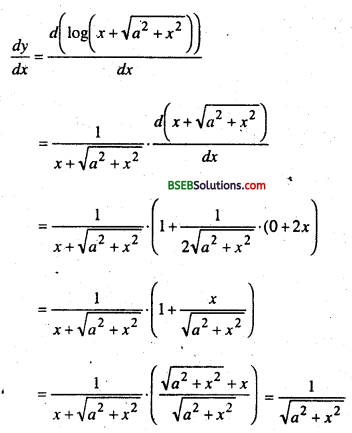(iii) Let y = $$\sqrt{\frac{1-\cos x}{1+\cos x}}$$ = log $$\sqrt{\frac{2-\sin ^{2} x / 2}{2 \cos ^{2} x / 2}}$$
or y = log $$\sqrt{\tan ^{2} x / 2}=\log \left(\tan \frac{x}{2}\right)$$
Diff w.r.to x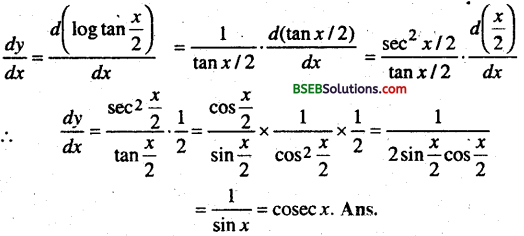(iv) Let y = log3 (log)xe
Diff w.r.to x
$$\frac{d y}{d x}=\frac{d\left(\log _{e}\left(\log _{e}^{x}\right)\right)}{d x}=\frac{1}{\log _{e}^{x}} \cdot \frac{1}{x}$$

(v) Let y = $$\tan ^{-1}\left(\frac{1-x}{1+x}\right)$$
Diff w.r.to x(vi) Let y = sin(x2 + 1)
Diff w.r.to x
$$\frac{d y}{d x}=\frac{d\left(\sin \left(x^{2}+1\right)\right)}{d x}=\cos \left(x^{2}+1\right) \cdot \frac{d\left(x^{2}+1\right)}{d x}$$
cos (x2 + 1).(2x + 0) = 2xcos (x2 + 1)

(vii) Let y = tan-1$$\frac{\sqrt{1+x^{2}-1}}{x}$$ put x = tan θ 1 + x2 = sec2Differentiability with respect for, we get
$$\frac{d y}{d x}=\frac{1}{2\left(1+x^{2}\right)}$$

(viii) Let y = (logx)x
⇒ log y = log x log (log x)
differentiablity w. r. for , we get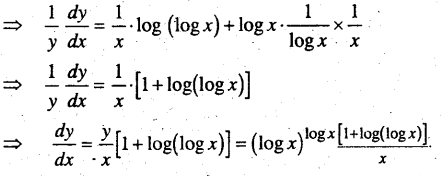(ix) Let y = xx = exlogex
With restpect to x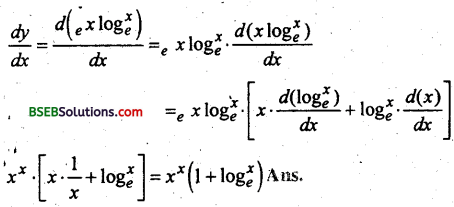(x) Let y = (log)x + xlogx
y = elogex + elogex. logex
Diff With respect to x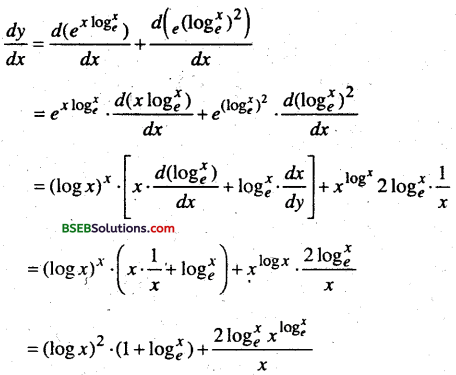(xi) Let y = cos (a cos x + bsin x)
⇒ $$\frac{d y}{d x}$$ = -sin (a cos x + b sin x_) $$\frac{d}{d x}$$ (acos x +b sin x)
= -sin(a cos x + b sin x ). (-a sin x + b cos x)

(xii) Let y = ecos-1x
cos-1x.loex
or y = e
Diff With respect to x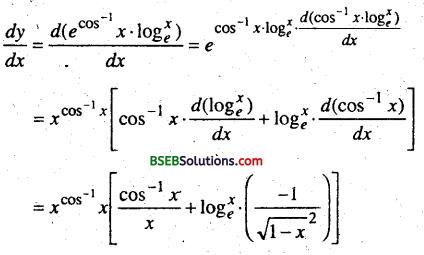(xiii) Let y = esinx + (tanx)x + (tan x)x = esinx + exloge tan x
Diff w.r.to xQuestion 2.
If y = sin(tan-12x) then prove that $$\frac{d y}{d x}=\frac{2}{\left(1+4 x^{2}\right)^{3 / 2}}$$
Solution:
Put tan-1 2x = t
y = sin(tan-12x) = sin t
$$\frac{d y}{d t}$$ = cos t
∴ tan -12x = t